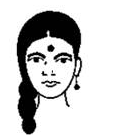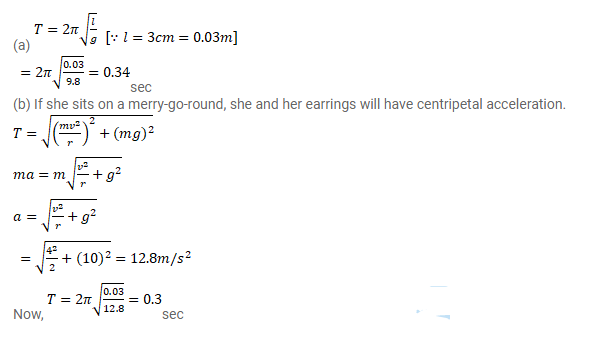# The ear ring of a lady shown in figure has a

Question:

The ear ring of a lady shown in figure has a $3 \mathrm{~cm}$ long light suspension wire. (a) Find the time period of small oscillations if the lady is standing on the ground. (b) The lady now sits in a merry-go-round moving at $4 \mathrm{~m} / \mathrm{s}$ in a circle of radius $2 \mathrm{~cm}$. Find the time period of small oscillations of the ear-ring.Solution: